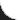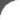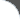Home | Tutorials | Articles | Videos | Products | Tools | Search
 Interviews | Open Source | Tag Cloud | Follow Us | Bookmark | ContactIn Browser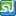StumbleUpon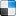del.icio.us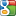Google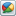Google Buzz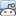reddit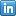LinkedIn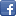Facebook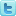TwitterLinkedin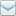E-Mail
 Tools and Libs > Uncommons Maths > Big Factorial

# Big Factorial

Uncommons Maths is a java library with nice math utilities and random number generators. This requires the library uncommons-maths-1.2.2.jar to be in classpath. The following example shows using Maths.bigFactorial() API.

 File Name  : com/bethecoder/tutorials/uncommons/BigFactorialTest.java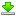Author  : Sudhakar KV Email  : [email protected]

 ``` package com.bethecoder.tutorials.uncommons; import java.math.BigInteger; import org.uncommons.maths.Maths; public class BigFactorialTest {   /**    * @param args    */   public static void main(String[] args) {          int num = 20;     BigInteger factorial = Maths.bigFactorial(num);     System.out.println("Factorial of " + num + " is " + factorial);          num = 30;     factorial = Maths.bigFactorial(num);     System.out.println("Factorial of " + num + " is " + factorial);          num = 40;     factorial = Maths.bigFactorial(num);     System.out.println("Factorial of " + num + " is " + factorial);          num = 50;     factorial = Maths.bigFactorial(num);     System.out.println("Factorial of " + num + " is " + factorial);   } }```

It gives the following output,
```Factorial of 20 is 2432902008176640000
Factorial of 30 is 265252859812191058636308480000000
Factorial of 40 is 815915283247897734345611269596115894272000000000
Factorial of 50 is 30414093201713378043612608166064768844377641568960512000000000000
```